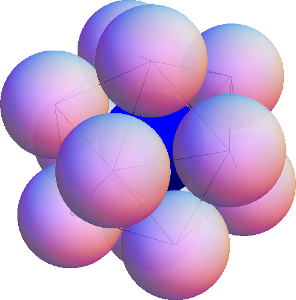# Kissing Spheres

If you put a quarter flat on a table, you can surround it with six other quarters that all touch the original. Is this the best you can do, if the coins can’t overlap? Well, yes: the coins are packed as tightly as possible, so there is no room for a seventh coin to fit around the middle one. We call this the Kissing Number Problem, and it gets much more interesting if you jump from two dimensions to three: given a sphere of radius 1, how many nonoverlapping spheres (of radius 1) can you place around it such that they all “kiss” the original sphere? Said differently, what is the kissing number in 3 dimensions?

Here’s a pretty good attempt: If you put one sphere at each vertex of a cuboctahedron like this,Twelve spheres all kissing a central (blue) sphere and arranged at the vertices of a cuboctahedron.

you’ll end up with 12 that seem pretty tightly packed. In another try, you could put a sphere at each vertex of an icosahedron:Twelve spheres all kissing a central (blue) sphere and arranged at the vertices of an icosahedron.

You’ll again get 12, but this time there is noticable wiggle room to move the spheres without losing contact with the central sphere—so much wiggle room, in fact, that any two spheres can switch places! Is there enough extra space for a 13th sphere to fit? This was a topic of heated debate between Isaac Newton and David Gregory (who believed 12 and 13, respectively), but it was not resolved until much later, in 1953, in favor of Newton’s 12. The proof is highly nontrivial.

What about still higher dimensions? What is the kissing number in four dimensions? (What does a 4-dimensional sphere even look like? Perhaps a topic of a future post…) This too is known, and the answer is 24—the proof is again quite difficult. See if you can figure out the arrangement! What about dimension 5, 6, 7, … ? As might be expected, the problem gets even harder in higher dimensions, and no other exact kissing numbers are known—except for two. Due to some veritable mathematical miracles (namely, the highly symmetric structures called the E8 lattice and the Leech lattice), the kissing numbers in dimensions 8 and 24 have been shown to be 240 and 196560, respectively.